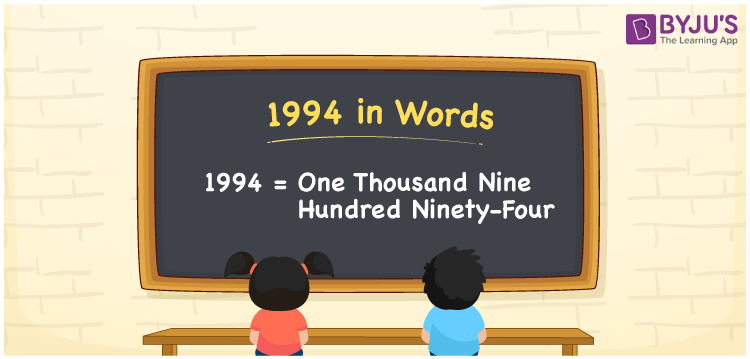# 1994 in Words

1994 in words is written as “One Thousand Nine Hundred Ninety-Four”. In Maths, 1994 is a cardinal number that expresses a quantity or a value. If the cost of a pair of jeans is Rs.1994, then it can be represented as Rupees One Thousand Nine Hundred Ninety-Four only. Learn more about Numbers In Words at BYJU’S and how to spell 1994 in words, in this article.

 1994 in Words One Thousand Nine Hundred Ninety-Four One Thousand Nine Hundred Ninety-Four in Numbers 1994

## 1994 in English Words## How to Write 1994 in Words?

The number 1994 in words can be written using a place value method. Since 1994 is a four-digit number, hence, the place value of each digit is given by;

 Thousands Hundreds Tens Ones 1 9 9 4

From the above table,

1 → Thousands place

9 → Hundreds place

9 → Tens place

4 → Ones place

So, when we read the number from right to left, it is spelt as One Thousand Nine Hundred Ninety-Four.

### Expanded Form of 1994

We can write the expanded form as:

1 x Thousand + 9 × Hundred + 9 × Ten + 4 × One

= 1 x 1000 + 9 × 100 + 9 × 10 + 4 × 1

= 1000 + 900 + 90 + 4

= 1994

= One Thousand Nine Hundred Ninety-Four

1994 is a whole number that is succeeded by 1993 and preceded by 1995. Learn more about the number 1994 below:

• 1994 in Words – One Thousand Nine Hundred Ninety-Four
• Is 1994 an odd number? – No
• Is 1994 an even number? – Yes
• Is 1994 a perfect square number? – No
• Is 1994 a perfect cube number? – No
• Is 1994 a prime number? – No
• Is 1994 a composite number? – Yes

## Frequently Asked Questions on 1994 in words

Q1

### What is 1994 in words?

1994 in words is given by One Thousand Nine Hundred Ninety-Four.
Q2

### What is the rule to write 1994 in words?

1994 in words is written based on the place values of each digit. 1 is at thousands, 9 at hundreds, 9 at tens and 4 at ones. Thus, reading from right to left, it is written as One Thousand Nine Hundred Ninety-Four.
Q3

### What is the value of 1900 plus 94 in words?

1900 + 94 = 1994 , i.e., One Thousand Nine Hundred Ninety-Four in words